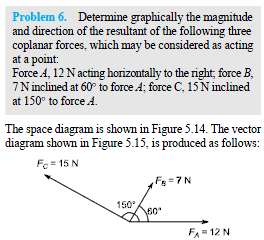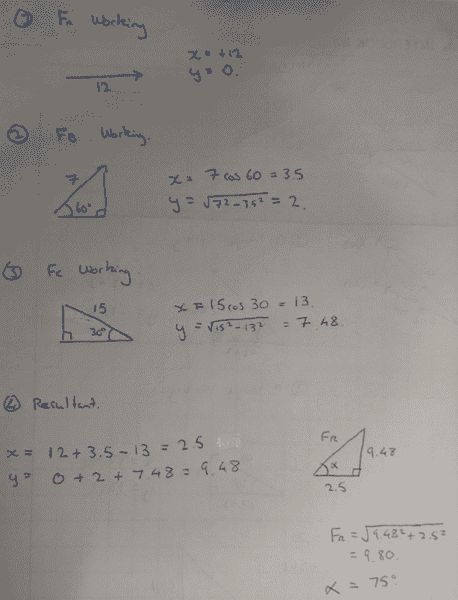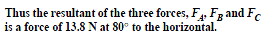# Calculating 3-Way Vectors

## Homework Statement## The Attempt at a Solution

I can't remember what this method of calculation this is as it's been a while since I've done this.[/B]

Could someone explain to me what I've done wrong in my calculations?

The answer was 13.8N at 80 degrees.Simon Bridge
Homework Helper
You are asked to do it graphically. The method you are looking for is "head to tail".
What you did was resolve the vectors into components, add the components... this approach is prone to compounding mistakes.
You probably have rounding errors due to irrational numbers, check your arithmetic too
i.e. the value for y in (2) is incorrect.

... notice
- for (2) and (3) the triangles are similar - in the technical sense: the 90-60-30 triangle has sides of ratios 1:2:√3
- force B and force C are 90deg to each other.

jedishrfu
Mentor
Look at how you computed y in the second vector is it really sqrt(7^2 - 3.5^2) = 2?

does 2^2 + 3.5^2 =?= 7^2? thats a simple check you could have done.

Also if you're going to use sin and cos why are you bothering with pythagoras? it takes longer to compute and its not elegant in this case.

The third vector y value is 15/2 = 7.5 an exact number which again shows why using Pythagoras causes a rounding problem that didn't need to be there.

You should KNOW that sin 60 = sqrt(3)/2 and cos 60 = 1/2 and you should know that sqrt(3) = 1.732...

Hope this helps.

Take care.

Last edited:
Thanks Simon and jedishrfu. It turns out it was the wrong calculation in (2) as you guys spotted.

Need to triple check my work next time•Simon Bridge